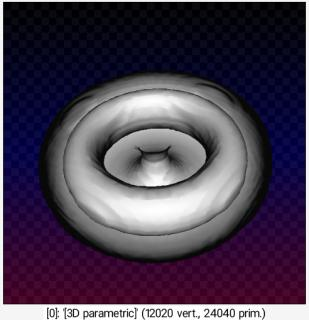Reference

 Table of Contents  ▸  List of Commands  ▸  3D Meshes  ▸  parametric3d ◀  opacity3d    |    pca_patch3d  ▶

# parametric3d

## Arguments:

• _x(a,b),_y(a,b),_z(a,b),_amin,_amax,_bmin,_bmax,_res_a>0,_res_b>0,_res_x>0,_res_y>0,_res_z>0,_smoothness>=0,_isovalue>=0

## Description:

Input 3D object from specified parametric surface (a,b) ⟶ (x(a,b),y(a,b),z(a,b)).

## Default values:

x=(2+cos(b))*sin(a), y=(2+cos(b))*cos(a), c=sin(b), amin=-pi, amax=pi, bmin=-pi, bmax=pi, res_a=512, res_b=res_a, res_x=64, res_y=res_x, res_z=res_y, smoothness=2% and isovalue=10%.

## Example of use:

\$ gmic parametric3d ,Command: parametric3d ,